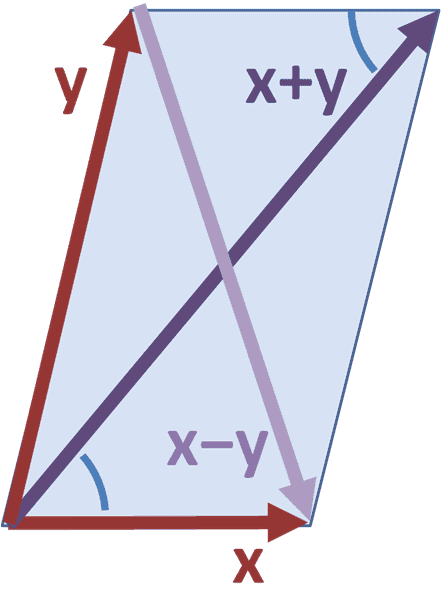# Symmetric Bilinear: What a Drag!

I am having problems showing the following:

##f## and ##g## are two linearly independent functions in ##E## and ##\theta : \mathbb{R} \to \mathbb{R}## is an additive map such that ##\theta(\mu \nu) = \theta(\mu)\nu +\mu \theta(\nu); \mu,\nu \in \mathbb{R}##. Show that the function;

##\psi \left(x\right)## ##=## ##f \left(x\right)\theta \left[g\left(x\right)\right]## ##-g\left(x\right)\theta\left[f\left(x\right)\right]##

satisfies the parallelogram property and the relation ##\psi\left(\lambda x\right)=\lambda ^{2}\psi\left(x\right)##.

Okay, so, I don't know where to start; I understand that I can begin to look at the picture of the parallelogram id. and show the similarities, like when the minus sign comes from. If you picture the two functions as vectors you can see that the minus sign comes from the direction in which the ##g## vector in directedand the same with ##f##, but after this, I cannot "show" it satisfies the second property.

PS: Think of ##\psi\left(x\right)## as being the inner product of ##x## with itself.

Last edited: